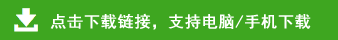# 人教版四年级下册数学第一单元《四则运算》测试题

42÷6＋43 =      9×8÷12 =      125－5×5 =
54－18＋9 =      48÷6×5 =      36×0＋64 =
0÷12÷6 =       35÷7×16 =     17＋0÷17 =

1、一个数加上（      ），还得原数；一个数和0相乘，得（      ）；0除以一个（         ）的数，还得0；（     ）不能作除数。

2、在计算（200-36×47）÷44时，要先算（               ）法，再算（          ）法，最后算（            ）法。